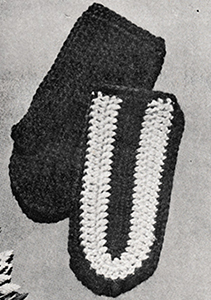## Crocheted Children's Mittens Pattern #120Printer-friendly versionSize: 5-8 years

MATERIAL:
1 skein Knitting Worsted (2 oz. skein)
Few yards contrasting color for trimming
1 bone crochet hook Size 5

GAUGE: 5 sts. = 1 inch

Palm: ch. 26
1st row: 1 s.c. in 2nd from hook, 1 s.c. in each st. to end of row, ch. 1, turn.
2nd row: incr. 1 st. in first st. (to increase a st. work 2 s.c. in 1 st.) 1 s.c. in each st. to end of row, ch. 1, turn.
3rd row: 1 s.c. in each st., increase 1 st. at end of row, ch. 1, turn.
4th row: increase 1 st. at beg. of row, 1 s.c. in each st. to end of row, ch. 1, turn.
5th row: 1 s.c. in each st., incr. 1 st. at end of row, ch. 1, turn.
6th row: 1 s.c. in each st., ch. 1, turn.
7th row: 1 s.c. in each st., ch. 1, turn.
8th row: 1 s.c. in each of the next 16 sts., ch. 10 for thumb, work 9 s.c. on thumb ch., 1 s.c. in each st. to end of row, ch. 1, turn.
9th row: work 1 s.c. in each of the next 21 sts., 2 s.c. in next st., (tip of thumb) 9 s.c. on foundation ch. of thumb, 1 s.c. in each st., decrease 1 st. in last st. (to decrease a st., draw up a loop in each of next 2 sts. and work them off as 1 st.), ch. 1, turn.
10th row: decrease 1 st. at beg. of row, work 1 s.c. in each st. to end of row, ch. 1, turn.
11th row: 1 s.c. in each st., decr. 1 st. at end of row, ch. 1, turn.
12th row: decr. 1 st. at beg. of row, 1 s.c. in each st. to end of row, ch. 1, turn. Fold thumb in half and sew up.
13th row: 1 s.c. in each st. omitting thumb. Break yarn, fasten off.
Back of Hand: ch. 24 with m.c.
1st round: 1 s.c. in 2nd st. from hook, 1 s.c. in each st., 3 s.c. in last st., 1 s.c. in each st. on other side of foundation ch., ch. 1, turn.
2nd round: 1 s.c. in each st., 3 s.c. in corner st., 1 s.c. in each st. to end of round; drop m.c., attach contrasting color, ch. 3, turn.
3rd round: 1 d.c. in each of the next 23 st., 2 d.c. in next st., 1 d.c. in next st., 2 d.c. in the following st., 1 d.c. in each st. to end of row (23 d.c.), ch. 3, turn.
4th round: 1 d.c. in each of the next 24 d.c., 2 d.c. in each of the next 3 sts., 1 d.c. in each st. to end of row (24 d.c.), drop contrasting color, pick up m.c., ch. 1, turn.
5th round: 1 s.c. in each of the next 24 sts., 2 s.c. in each of the next 6 sts., 1 s.c. in each of the next 24 sts. Break yarn, fasten off.
Finishing: Crochet mitten tog. with 1 row s.c. on right side with m.c. Crochet 2 rows s.c. (26 s.c. in each round) around wrist in m.c.